# How to Set, List and Manage Linux Environment Variables

March 22nd, 2022Environment variables are a key aspect of Linux administration and provide engineers with a powerful tool for customizing systems. In this article, we’ll take a closer look at what Linux environment variables are, how they differ from shell variables, and how you can list, set, and manage them.

## #What are Linux Environment Variables?

Linux environment variables are dynamic variables used by a shell and its child processes. Environment variables define a variety of aspects related to how a Linux system works. For example, a user’s default shell is defined in the SHELL variable. Similarly, many administrators use the JAVA_HOME= environment variable to point programs to specific versions of Java on a system.

A few key points to keep in mind when working with Linux environment variables are:

• Environment variables follow `<NAME>=<VALUE>` formatting.
• You can specify multiple values for a single variable by separating them with colons like this:
`<NAME>=<VALUE1>:<VALUE2>:<VALUE3>`
• Environment variables are case-sensitive.
• By convention, but not rule, environment variable names are always capitalized.
• Shell variables are not the same as environment variables. Shell variables only apply to the current shell, not any child processes. We’ll take a closer look at this topic in the Linux shell variables vs. Linux environment variables section.

With all that in mind, let’s move on to the tutorial.

## #Prerequisites

Our examples use Ubuntu 20.04 and GNU Bash 5.0.17, but you can follow along on most modern Linux systems.
How to list Linux environment variables
There are multiple methods for listing Linux environment variables here we will demonstrate some of the most common.

### #Use printenv to List Linux Environment Variables

The `printenv` command displays the current environment variables.

You can use `printenv` to list all your current Linux environment variables by running the command without any options or parameters.

``````printenv
``````

The output of should look similar to this:

``````SHELL=/bin/bash
SUPERBREAKFAST=Pepper and Egg
PWD=/root
LOGNAME=root
XDG_SESSION_TYPE=tty
MOTD_SHOWN=pam
HOME=/root
LANG=C.UTF-8
LS_COLORS=rs=0:di=01;34:ln=01;36:mh=00:pi=40;33:so=01;35:do=01;35:bd=40;33;01:cd=40;33;01:or=40;31;01:mi=00:su=37;41:sg=30;43:ca=30;41:tw=30;42:ow=34;42:st=37;44:ex=01;32:*.tar=01;31:*.tgz=01;31:*.arc=01;31:*.arj=01;31:*.taz=01;31:*.lha=01;31:*.lz4=01;31:*.lzh=01;31:*.lzma=01;31:*.tlz=01;31:*.txz=01;31:*.tzo=01;31:*.t7z=01;31:*.zip=01;31:*.z=01;31:*.dz=01;31:*.gz=01;31:*.lrz=01;31:*.lz=01;31:*.lzo=01;31:*.xz=01;31:*.zst=01;31:*.tzst=01;31:*.bz2=01;31:*.bz=01;31:*.tbz=01;31:*.tbz2=01;31:*.tz=01;31:*.deb=01;31:*.rpm=01;31:*.jar=01;31:*.war=01;31:*.ear=01;31:*.sar=01;31:*.rar=01;31:*.alz=01;31:*.ace=01;31:*.zoo=01;31:*.cpio=01;31:*.7z=01;31:*.rz=01;31:*.cab=01;31:*.wim=01;31:*.swm=01;31:*.dwm=01;31:*.esd=01;31:*.jpg=01;35:*.jpeg=01;35:*.mjpg=01;35:*.mjpeg=01;35:*.gif=01;35:*.bmp=01;35:*.pbm=01;35:*.pgm=01;35:*.ppm=01;35:*.tga=01;35:*.xbm=01;35:*.xpm=01;35:*.tif=01;35:*.tiff=01;35:*.png=01;35:*.svg=01;35:*.svgz=01;35:*.mng=01;35:*.pcx=01;35:*.mov=01;35:*.mpg=01;35:*.mpeg=01;35:*.m2v=01;35:*.mkv=01;35:*.webm=01;35:*.ogm=01;35:*.mp4=01;35:*.m4v=01;35:*.mp4v=01;35:*.vob=01;35:*.qt=01;35:*.nuv=01;35:*.wmv=01;35:*.asf=01;35:*.rm=01;35:*.rmvb=01;35:*.flc=01;35:*.avi=01;35:*.fli=01;35:*.flv=01;35:*.gl=01;35:*.dl=01;35:*.xcf=01;35:*.xwd=01;35:*.yuv=01;35:*.cgm=01;35:*.emf=01;35:*.ogv=01;35:*.ogx=01;35:*.aac=00;36:*.au=00;36:*.flac=00;36:*.m4a=00;36:*.mid=00;36:*.midi=00;36:*.mka=00;36:*.mp3=00;36:*.mpc=00;36:*.ogg=00;36:*.ra=00;36:*.wav=00;36:*.oga=00;36:*.opus=00;36:*.spx=00;36:*.xspf=00;36:
SSH_CONNECTION=24.148.23.154 2068 84.32.188.154 22
EASTEREGG=You pay attention to detail, good job!
LESSCLOSE=/usr/bin/lesspipe %s %s
XDG_SESSION_CLASS=user
TERM=xterm
LESSOPEN=| /usr/bin/lesspipe %s
USER=root
SHLVL=1
XDG_SESSION_ID=839
XDG_RUNTIME_DIR=/run/user/0
SSH_CLIENT=24.148.23.154 2068 22
XDG_DATA_DIRS=/usr/local/share:/usr/share:/var/lib/snapd/desktop
PATH=/usr/local/sbin:/usr/local/bin:/usr/sbin:/usr/bin:/sbin:/bin:/usr/games:/usr/local/games:/snap/bin
SSH_TTY=/dev/pts/0
_=/usr/bin/printenv
``````

You can also use `printenv` to display individual environment variables. The basic syntax to display a single Linux environment variable with `printenv` is:
`printenv [name]`

For example, to print the `PWD` environment variable, use this command:

``````printenv PWD
``````

The output should display your shell’s current working directory.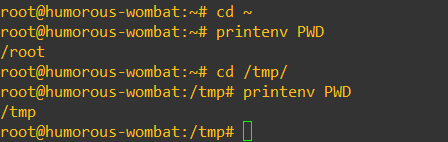### #Use env to List Linux Environment Variables

The env command can list all environment variables with output similar to `printenv`. To use env to list all your Linux environment variables, run the command with no options or parameters, like this:

``````env
``````

In most cases, the `env` output should match the output of `printenv` except for the `_=` variable. This happens because the `_=` variable is a special Bash parameter used to invoke a shell or shell script. If you use env on a modern Linux system, the `_=` value will match the location of the `env` binary (e.g. /usr/bin/env). If you use `printenv`, it will match the location of the printenv binary (e.g./usr/bin/printenv).

### #Use echo to Display a Linux Environment Variable

One of the quickest ways to display a single Linux environment variable is to use the `echo` command. The basic syntax is `echo \$<variable_name>`.

For example, to print the PATH environment variable, use this command:

``````echo \$PATH
``````

The output should look similar to:

``````/usr/local/sbin:/usr/local/bin:/usr/sbin:/usr/bin:/sbin:/bin:/usr/games:/usr/local/games:/snap/bin
``````

The `echo \$<variable_name>` command also works for shell variables that are NOT environment variables. For example, if we create a BREAKFAST variable and set it to 'Pepper & Egg', we can see the output with `echo \$BREAKFAST` but not with `printenv`.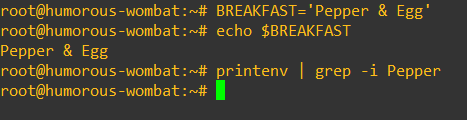### #Other Methods to List Linux Environment Variables

Other methods for listing Linux environment variables include:

• `declare` - this built-in shell utility can display shell and environment variables using this command.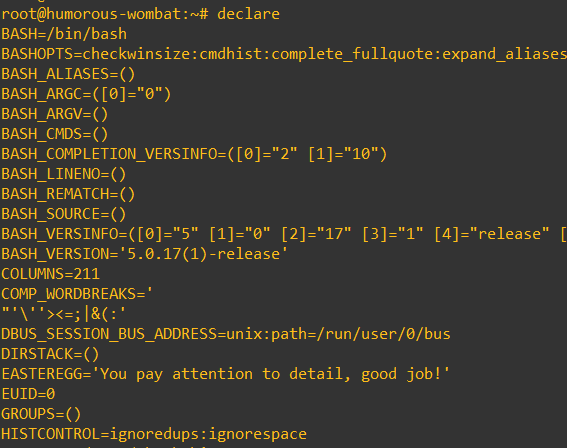• `set` - displays shell variables, environment variables, and shell functions.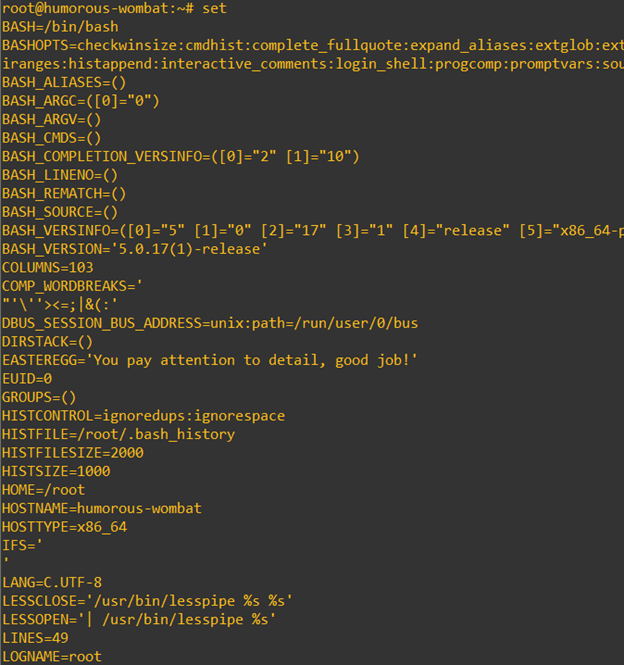• `compgen -v` - displays the names of all your shell and environment variables.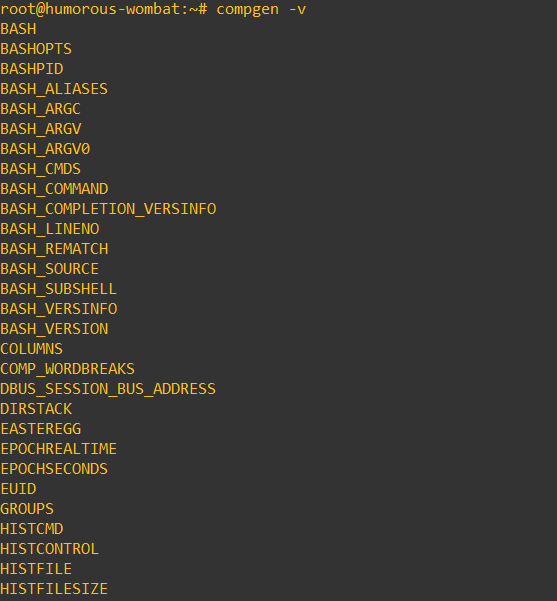## #How to set Linux Environment Variables

The basic command to set a Linux environment variable is

``````export <NAME>='<value>'
``````

For example, to create an environment variable named SANDWICH with a value of Pepper And Egg, use this command:

``````export SANDWICH='Pepper And Egg'
``````

To confirm that the environment variable was created, use the command

``````printenv SANDWICH
``````

The output should be as follows: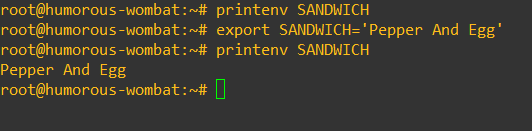### #How to Create a Shell Variable

You can create shell variables using this basic command structure `<NAME>='<value>'`.

For example, to create a shell variable named COOLSERVER with a value of Cherry, use this command:

``````COOLSERVER='Cherry'
``````

You can confirm the variable now exists using this command:

``````echo \$COOLSERVER
``````

The output should be:

``````Cherry
``````

To confirm the variable is not an environment variable, execute this command:

``````printenv COOLSERVER
``````

Because the variable isn’t an environment variable, there should be no output: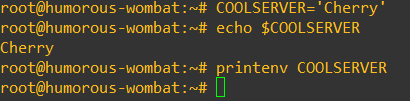### #How to Convert a Shell Variable to an Environment Variable

To convert a shell variable to an environment variable, the basic command syntax is `export <Shell_Variable_Name>`.

For example, to convert the COOLSERVER variable from the previous example to an environment variable, use this command:

``````export COOLSERVER
``````

Now, the `printenv COOLSERVER` command should print Cherry as the output: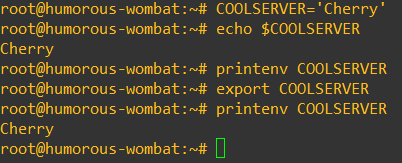### #Linux Shell Variables vs. Linux Environment Variables

With what we have covered so far, you can see shell variables and environment variables are different things. Now, let’s take a closer look at the difference and why it matters.

The key difference between Linux shell variables and Linux environment variables is:
shell variables are not shared with a shell’s child processes, environment variables are shared with a shell's child processes.

To demonstrate why that difference is important, let’s walk through an example with a simple bash script.

First, create a variable named CLOUD and set the value to Cherry Servers!.

``````CLOUD='Cherry Servers!'
``````

Next, use the `echo` command to display the variable.

``````echo \$CLOUD
``````

Here’s what that looks like end-to-end:Now, create a `test.sh` bash script that includes the same command. The script should look like this:

``````#!/bin/bash
echo \$CLOUD
``````

Make the script executable with this command:

``````chmod +x test.sh
``````

Now, run the script:

``````./test.sh
``````

The output should be blank, like this: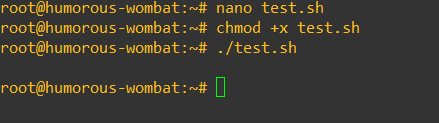The reason the output is blank is because `\$CLOUD` is a shell variable. It is contained within the current shell instance. Meanwhile, the `test.sh` script initiates a new process,and, since the `\$CLOUD` environment variable is not set, the script doesn’t print anything.

Let’s make `\$CLOUD` an environment variable with this command (note do NOT use the \$ before the variable name):

``````export CLOUD
``````

Now, run the test.sh script again.

``````./test.sh
``````

You should see this output: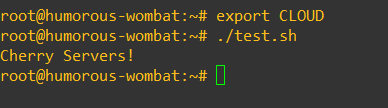## #How to Delete Linux Environment Variables

The basic syntax to delete an existing Linux environment variable is `unset <NAME>`.

For example, to unset our COOLSERVER variable, use this command:

``````unset COOLSERVER
``````

To confirm the variable is now deleted, execute this command:

``````printenv COOLSERVER
``````

Because the variable isn’t an environment variable, there should be no output: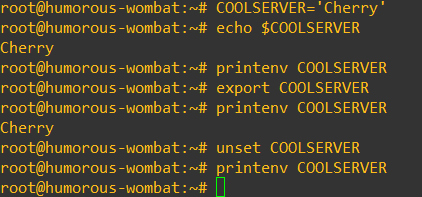## #How to Make Linux Environment Variables Permanent

The environment variables we’ve created so far will only last for the duration of your shell session. If you log out or reboot, you would need to recreate them if you want to use them.

Fortunately, there are several ways to make Linux environment variables persist across different shell sessions.

### #Use .profile to Make Environment Variables Permanent for Login Shells

The `.profiile` file exists in a user’s home directory. You can add environment variables for a user by editing their `.profile` file to include export commands to set environment variables.

For example, to make our COOLSERVER environment variable permanent for our current user, follow this process:

1. Change directory to the user’s home directory `cd ~`
2. Open `.profile` file in a text editor (e.g. nano, vi, vim, etc.)
3. Add the following line to the bottom of the file: `export COOLSERVER='Cherry'`
4. Save the changes

The changes will take effect on the next login. You can make them take effect for the current terminal using this command:

``````. ~/.profile
``````

### #Use .bashrc to Make Environment Variables Permanent for Non-login Interactive Shells

For non-login shells, you can follow the same process as login shells, but instead of editing `~/.profile` file, edit `~/.bashrc`.

### #Use /etc/environment to Make Environment Variables Permanent System-wide

⚠️ Warning: Any shell on the system can access system-wide variables, so set them with caution.

To set system-wide Linux environment variables, you can edit the `/etc/environment` file. Instead of appending export commands to the file, append the `<NAME>='<VALUE>'` pair to the end of the file.

For example, to make our COOLSERVER environment variable permanent for the entire system, follow this process:

1. Change directory to the user’s home directory `cd /etc`
2. Open `environment` file in a text editor (e.g. nano, vi, vim, etc.)
3. Add the following line to the bottom of the file `COOLSERVER='Cherry'`
4. Save the changes

## #Next Steps with Linux Environment Variables

Now that you know how to list, set, and manage Linux environment variables, you can use them to customize your systems and scripts. To take a deeper dive on some of the topics we reviewed here, check out the man pages for bash, export, and env.

For more content like this, subscribe to the Cherry Servers blog!

Helping engineers learn 💡 about new technologies and ingenious IT automation use cases to build better systems 💻

#### Join Cherry Servers Community

Get monthly practical guides about building more secure, efficient and easier to scale systems on an open cloud ecosystem.